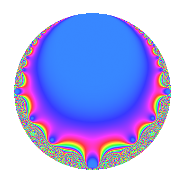# Properties

 Label 7.5.bLevel 7 Weight 5 Character orbit b Rep. character $$\chi_{7}(6,\cdot)$$ Character field $$\Q$$ Dimension 1 Newforms 1 Sturm bound 3 Trace bound 0

# Related objects

## Defining parameters

 Level: $$N$$ = $$7$$ Weight: $$k$$ = $$5$$ Character orbit: $$[\chi]$$ = 7.b (of order $$2$$ and degree $$1$$) Character conductor: $$\operatorname{cond}(\chi)$$ = $$7$$ Character field: $$\Q$$ Newforms: $$1$$ Sturm bound: $$3$$ Trace bound: $$0$$

## Dimensions

The following table gives the dimensions of various subspaces of $$M_{5}(7, [\chi])$$.

Total New Old
Modular forms 3 3 0
Cusp forms 1 1 0
Eisenstein series 2 2 0

## Trace form

 $$q$$ $$\mathstrut +\mathstrut q^{2}$$ $$\mathstrut -\mathstrut 15q^{4}$$ $$\mathstrut +\mathstrut 49q^{7}$$ $$\mathstrut -\mathstrut 31q^{8}$$ $$\mathstrut +\mathstrut 81q^{9}$$ $$\mathstrut +\mathstrut O(q^{10})$$ $$q$$ $$\mathstrut +\mathstrut q^{2}$$ $$\mathstrut -\mathstrut 15q^{4}$$ $$\mathstrut +\mathstrut 49q^{7}$$ $$\mathstrut -\mathstrut 31q^{8}$$ $$\mathstrut +\mathstrut 81q^{9}$$ $$\mathstrut -\mathstrut 206q^{11}$$ $$\mathstrut +\mathstrut 49q^{14}$$ $$\mathstrut +\mathstrut 209q^{16}$$ $$\mathstrut +\mathstrut 81q^{18}$$ $$\mathstrut -\mathstrut 206q^{22}$$ $$\mathstrut -\mathstrut 734q^{23}$$ $$\mathstrut +\mathstrut 625q^{25}$$ $$\mathstrut -\mathstrut 735q^{28}$$ $$\mathstrut +\mathstrut 1234q^{29}$$ $$\mathstrut +\mathstrut 705q^{32}$$ $$\mathstrut -\mathstrut 1215q^{36}$$ $$\mathstrut -\mathstrut 1294q^{37}$$ $$\mathstrut -\mathstrut 334q^{43}$$ $$\mathstrut +\mathstrut 3090q^{44}$$ $$\mathstrut -\mathstrut 734q^{46}$$ $$\mathstrut +\mathstrut 2401q^{49}$$ $$\mathstrut +\mathstrut 625q^{50}$$ $$\mathstrut -\mathstrut 5582q^{53}$$ $$\mathstrut -\mathstrut 1519q^{56}$$ $$\mathstrut +\mathstrut 1234q^{58}$$ $$\mathstrut +\mathstrut 3969q^{63}$$ $$\mathstrut -\mathstrut 2639q^{64}$$ $$\mathstrut +\mathstrut 4946q^{67}$$ $$\mathstrut +\mathstrut 2914q^{71}$$ $$\mathstrut -\mathstrut 2511q^{72}$$ $$\mathstrut -\mathstrut 1294q^{74}$$ $$\mathstrut -\mathstrut 10094q^{77}$$ $$\mathstrut -\mathstrut 3646q^{79}$$ $$\mathstrut +\mathstrut 6561q^{81}$$ $$\mathstrut -\mathstrut 334q^{86}$$ $$\mathstrut +\mathstrut 6386q^{88}$$ $$\mathstrut +\mathstrut 11010q^{92}$$ $$\mathstrut +\mathstrut 2401q^{98}$$ $$\mathstrut -\mathstrut 16686q^{99}$$ $$\mathstrut +\mathstrut O(q^{100})$$

## Decomposition of $$S_{5}^{\mathrm{new}}(7, [\chi])$$ into irreducible Hecke orbits

Label Dim. $$A$$ Field CM Traces $q$-expansion
$$a_2$$ $$a_3$$ $$a_5$$ $$a_7$$
7.5.b.a $$1$$ $$0.724$$ $$\Q$$ $$\Q(\sqrt{-7})$$ $$1$$ $$0$$ $$0$$ $$49$$ $$q+q^{2}-15q^{4}+7^{2}q^{7}-31q^{8}+3^{4}q^{9}+\cdots$$